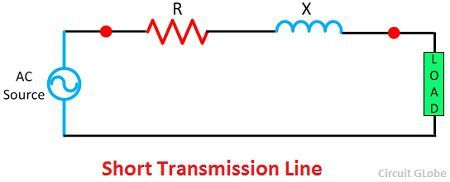# Classification of Transmission Lines

The classification of the transmission lines depends on its voltage and the length of the conductor. The transmission line is the medium of transferring the power from the generating station to the load centre. It is mainly classified into two types. They are the

1. AC Transmission Line
• Short-Transmission Line
• Medium Transmission Line
• Pi Model of a Medium Transmission Line
• T Model of a Medium Transmission Line
• Long Transmission Line
2. DC Transmission Line

## 1. AC Transmission Line

The transmission line has resistance R, inductance L, capacitance C and the shunt or leakage conductance G. These parameters along with the load and the transmission line determine the performance of the line. The term performance means the sending end voltage, sending end currents, sending end power factor, power loss in the line, efficiency of the transmission line, regulate and limit of power flow during efficiency and transmission, regulation and limits of power during steady state and transient condition. The comparison chart of the transmission line is shown in the figure below.## Short Transmission Line

If the line is not more than 80 KV or if the voltage is not over than 66 KV then the line is known as the short transmission line. The capacitance of the line is governed by their length. The effect of capacitance on the short transmission line is negligible, but for cable where the distance between the conductor is small, the effect of capacitance cannot be ignored. While studying the performance of the short transmission line only resistance and the inductance of the line is calculated.## Medium Transmission Line

The line which is ranging from 80 to 240 km is termed as a medium transmission line. The capacitance of the medium transmission line cannot be ignored. The capacitance of the medium transmission line is considered to be lumped at one or more point of the lines. The effect of the line is more at high frequency, and their leakages inductance and capacitance is considered to be neglected. The medium transmission line is sub-divided into Pi – model and T – model.

### Pi Model of a Medium Transmission Line

In nominal Pi model, it is assumed that the half of the capacitance concentrate at the each end of the line.### T – Model of a Medium Transmission Line

In T model, it is assumed that the capacitance is concentrated at the centre of the line.## Long Transmission Line

The line having a length more than 240 km is considered a long transmission line. All the four parameters (resistance, inductance, capacitance, and leakage conductance) are found to be equally distributed along the entire length of the line.## 2. DC Transmission Line

The DC transmission is mainly used for the bulk power transmission. For long distance transmission, the DC is less expensive and have low electrical losses. The cost of the DC transmission systems is higher for short distance transmission line because it requires more convertible equipment as compared to an AC system.The converter station converts the AC to DC at the sending end and DC to AC at the load end of the line. One of the major advantage of the DC system is that it allows the power transmission between two unsynchronised AC system.

### 3 thoughts on “Classification of Transmission Lines”

1. You have explained the topic very well but I have a confusion about it. I could not understand the purpose of doing this classification.